# How many 15

How many six-eights are there in 18? Which of the following would you choose to solve this question?
18 x 6/8 or 18 : 6/8

n =  24

### Step-by-step explanation:

$n=18\mathrm{/}\frac{6}{8}=24$Did you find an error or inaccuracy? Feel free to write us. Thank you!Tips to related online calculators
Need help to calculate sum, simplify or multiply fractions? Try our fraction calculator.

## Related math problems and questions:

• Simplest form of a fractionWhich one of the following fraction after reducing in simplest form is not equal to 3/2? a) 15/20 b) 12/8 c) 27/18 d) 6/4
• Dividing walnuts into crates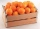There are 8 and 2 over 3 pounds of walnuts in a container, which will be divided equally into containers that hold 1 and 1 over 5 pounds. This would fill n and 4 over 18 containers. What is n?
• Shirts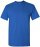In a classroom,1/6 of the students are wearing blue shirts, and 2/3 are wearing white shirts. There are 18 students in the classroom. How many students are wearing shirts other than blue shirts or white shirts?
• JonahJonah has an 8-pound bag of potting soil. He divides it evenly among 5 flowerpots. How much soil is in each pot? Show your answer as a fraction or mixed number. Solve this problem any way you choose.
• A ropeA rope can be cut into equal length with no rope left over. The lengths can be 15cm,18cm or 25cm. What is the shortest possible length of the rope?
• DigitsHow many odd four-digit numbers can we create from digits: 0, 3, 5, 6, 7? (a) the figures may be repeated (b) the digits may not be repeated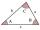The size of two internal angles of a triangle ABC are α=6/18π and β=7/18π. Calculate the size of the third angle.
• To improper fractionChange mixed number to improper fraction a) 1 2/15 b) -2 15/17
• Giraffes to monkeysThe ratio of the number of giraffes to the number of monkeys in a zoo is 2 to 5. Which statement about the giraffes and monkeys could be true? A. For every 10 monkeys in the zoo, there are 4 giraffes. B. For every  giraffe in the zoo, there are 3 monkeys.
• Six-eightsSix-eights of the one hundred pupils joined the Math Glee club. If the Math Glee club members were grouped into three, how many members were in each group?
• Golf ballsOf the 28 golf balls, 1/7 are yellow. How many golf balls are yellow? Use the model to help you. Enter your answer in the box.
• Sally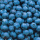Sally is making 15 individual blueberry cheesecakes. How many pounds of blueberries will be in each cheesecake if 5 1/4 pounds of blueberries are divided equally among them?
• Equation 15Solve equation with variables on both sides:
• PlumsIn the bowl are plums. How many would be there if we can divide it equally among 8, 10 and 11 children?
• Denissa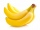Denissa has 18 9/10 kilos of bananas to sell if she was able to sell 15 ⅗. How many kilos of bananas were left?
• Four paversFour pavers would pave the square in 18 days. How many pavers do you need to add to done work in 12 days?
• How many 16How many three-tenths are there in two and one-fourths?